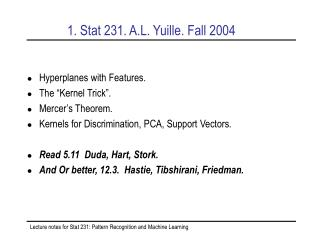# 1. Stat 231. A.L. Yuille. Fall 2004 - PowerPoint PPT PresentationDownload Presentation1. Stat 231. A.L. Yuille. Fall 2004

1. Stat 231. A.L. Yuille. Fall 2004Download Presentation## 1. Stat 231. A.L. Yuille. Fall 2004

- - - - - - - - - - - - - - - - - - - - - - - - - - - E N D - - - - - - - - - - - - - - - - - - - - - - - - - - -
##### Presentation Transcript

1. 1. Stat 231. A.L. Yuille. Fall 2004 • Hyperplanes with Features. • The “Kernel Trick”. • Mercer’s Theorem. • Kernels for Discrimination, PCA, Support Vectors. • Read 5.11 Duda, Hart, Stork. • And Or better, 12.3. Hastie, Tibshirani, Friedman. Lecture notes for Stat 231: Pattern Recognition and Machine Learning

2. 2. Beyond Linear Classifiers • Increase the dimensions of the data using Feature Vectors. • Search for a linear hyperplane between features • Logical X-OR. • X-OR requires decision rule • Impossible with a linear classifer. • Define • Then solve XOR by hyperplane Lecture notes for Stat 231: Pattern Recognition and Machine Learning

3. 3. Which Feature Vectors? • With sufficient feature vectors we can perform any classification using the linear separation algorithms applied to feature space. • Two Problems: 1. How to select the features? 2. How to achieve Generalization and prevent overlearning? • The Kernel Trick simplifies both problems. (But we won’t address (2) for a few lectures). Lecture notes for Stat 231: Pattern Recognition and Machine Learning

4. 4. The Kernel Trick • Kernel Trick: Define the kernel: • Claim: linear separation algorithms in feature space only depends on • Claim:we can use all results from linear separation (previous two lectures) by replacing all dot-products Lecture notes for Stat 231: Pattern Recognition and Machine Learning

5. 5. The Kernel Trick • Hyperplanes in feature space are surfaces for • With associated classifier • Determine the classifier that maximizes the margin, as in previous lecture, replacing • The dual problem depends only onby the dot products • Replace them by Lecture notes for Stat 231: Pattern Recognition and Machine Learning

6. The Kernel Trick • Solve the dual to get the which depends only on K. • Then the solution is: Lecture notes for Stat 231: Pattern Recognition and Machine Learning

7. 6. Learning with Kernels • All the material in the previous lecture can be adapted directly By replacing the dot product by the kernel • Margins, Support Vectors, Primal and Dual Problems. • Just specify the kernel, don’t bother with the features • The kernel trick depends on the quadratic nature of the learning problem. It can be applied to other quadratic problems, eg. PCA. Lecture notes for Stat 231: Pattern Recognition and Machine Learning

8. 7. Example Kernels • Popular kernels are • Constants: • What conditions, if any, need we put on kernels to ensure that they can be derived from features? Lecture notes for Stat 231: Pattern Recognition and Machine Learning

9. 8. Kernels, Mercer’s Theorem • For a finite dataset express kernel as a matrix with components • The matrix is symmetric and positive definite matrix with eigenvalues and eigenvectors • Then • Feature vectors: Lecture notes for Stat 231: Pattern Recognition and Machine Learning

10. 9. Kernels, Mercer’s Theorem • Mercer’s Theorem extends this result to • Functional Analysis (F.A). Most results in Linear Algebra can be extended to F.A. (Matrices with infinite dimensions). • E.G. We define eigenfunctions of requiring finite • Provided is positive definite, the features are Almost any kernel is okay. Lecture notes for Stat 231: Pattern Recognition and Machine Learning

11. 10. Kernel Examples. • Figure of kernel discrimination. Lecture notes for Stat 231: Pattern Recognition and Machine Learning

12. 11. Kernel PCA • The kernel trick can be applied to any quadratic problem. • PCA: Seek eigenvectors and eigenvalues of • Where, wlog • In feature space, replace • All non-zero eigenvectors are of form • Reduces to solving • Then Lecture notes for Stat 231: Pattern Recognition and Machine Learning

13. 12.Summary. • The Kernel Trick allows us to do linear separation in feature space. • Just specify the kernel, no need to explicitly specify the features. • Replace dot product with the kernel. • Allows classifications impossible using linear separation on original features Lecture notes for Stat 231: Pattern Recognition and Machine Learning